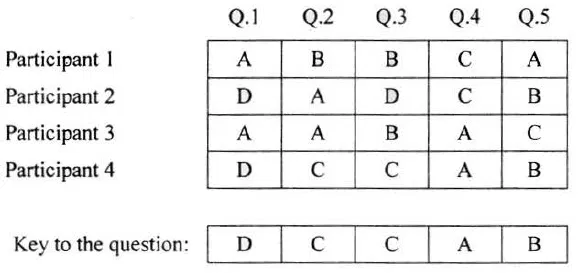# Java program to accept user response of quiz and answer key and find the result

The result of a quiz competition is to be prepared as follows:

The quiz has five questions with four multiple choices (A, B, C, D), with each question carrying 1 mark for the correct answer. Design a program to accept the number of participants N such that N must be greater than 3 and less than 11. Create a double dimensional array of size (Nx5) to store the answers of each participant row-wise.

Calculate the marks for each participant by matching the correct answer stored in a single dimensional array of size 5. Display the scores for each participant and also the participant(s) having the highest score.

Example: If the value of N = 4, then the array would be:Test your program with the sample data and some random data:

Example 1

INPUT : N = 5

Participant 1 D A B C C

Participant 2 A A D C B

Participant 3 B A C D B

Participant 4 D A D C B

Participant 5 B C A D D

Key: B C D A A

OUTPUT : Scores :

Participant 1 D A B C C

Participant 1 = 0

Participant 2 = 1

Participant 3 = 1

Participant 4 = 1

Participant 5 = 2

Highest score: Participant 5

Example 2

INPUT : N = 4

Participant 1 A C C B D

Participant 2 B C A A C

Participant 3 B C B A A

Participant 4 C C D D B

Key: A C D B B

OUTPUT : Scores :

Participant 1 = 3

Participant 2 = 1

Participant 3 = 1

Participant 4 = 3

Highest score:

Participant 1

Participant 4

Example 3

INPUT : N = 12

OUTPUT : INPUT SIZE OUT OF RANGE.

`Solution`

``````import java.util.*;
class QuizResult
{
char A[][],K[];
int S[],n;

void input()
{
Scanner sc = new Scanner(System.in);
System.out.print("Enter number of participants : ");
n = sc.nextInt();
if(n<4 || n>10)
{
System.out.println("INPUT SIZE OUT OF RANGE");
System.exit(0);
}
A = new char[n]; // Array to store the answers of every participants
K = new char; // Array to store answer key
S = new int[n]; // Array to store score of every participant
System.out.println("\n* Enter answer of each participant row-wise in a single line *\n");
for(int i = 0; i<n; i++)
{
System.out.print("Participant "+(i+1)+" : ");
for(int j=0; j<5; j++)
{
A[i][j] = sc.next().charAt(0);
}
}
for(int i = 0; i<5; i++)
{
K[i] = sc.next().charAt(0);
}
}

void CalcScore() // Function to calculate score of every participant
{

for(int i = 0; i<n; i++)
{
S[i] = 0;
for(int j=0; j<5; j++)
{
if(A[i][j] == K[j]) // Checking if Answer of the participants match with the key or not
{
S[i]++;
}
}
}
}

void printScore()
{
int max = 0;
System.out.println("\nSCORES : ");
for(int i = 0; i<n; i++)
{
System.out.println("\tParticipant "+(i+1)+" = "+S[i]);
if(S[i]>max)
{
max = S[i]; // Storing the Highest Score
}
}
System.out.println();

System.out.println("\tHighest Score : "+max);

System.out.println("\tHighest Scorers : ");
for(int i = 0; i<n; i++) // Printing all those participant number who got highest score
{
if(S[i] == max)
{
System.out.println("\t\t\tParticipant "+(i+1));
}
}
}

public static void main(String args[])
{
QuizResult ob = new QuizResult();
ob.input();
ob.CalcScore();
ob.printScore();
}
}
``````
`Output`

Enter number of participants : 4

* Enter answer of each participant row-wise in a single line *

Participant 1 : A C C B D

Participant 2 : B C A A C

Participant 3 : B C B A A

Participant 4 : C C D D B